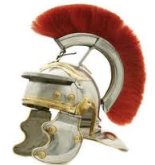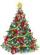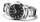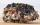Added together and write as decimal number:

LXVII + MLXIV

LXVII + MLXIV =  1131

### Step-by-step explanation:

$LXVII+MLXIV=67+1064=1131$Did you find an error or inaccuracy? Feel free to write us. Thank you!## Related math problems and questions:

• Roman numerals +Add up the number written in Roman numerals. Write the results as a decimal number.
• Roman numerals 2+Add up the number written in Roman numerals. Write the results as roman numbers.
• Roman numeralsWrite numbers written in Roman numerals as decimal.
• Roman numerals 2-Subtract up the number written in Roman numerals. Write the results as Roman numbers.
• ClassmatesRoman is ranked 12th highest and eleventh lowest pupil. How many classmates does Roman have?Add and write the result again as hours, minutes, seconds: 2hodiny45min15s + 1h20m50s =
• FractionsSort fractions z1 = (6)/(11); z2 = (10)/(21); z3 = (19)/(22) by its size. Result write as three serial numbers 1,2,3.
• ChristmasCalculate how much of the school year (202 days long) take Christmas holidays 19 days long. Expressed as a decimal number and as a percentage.
• MinutesWrite as fraction in basic form which part of the week is 980 minutes.
• BusOn the 4-th stop take on 56 and take off 38 passengers. How many were added (write as positive number) or shrunk (write as negative number) the count of passengers?
• Expression 1What is 7+8-(5×2)+5-4+(6×(5-3)+6)-(8+10)-7+6?
• Fraction and a decimalWrite as a fraction and a decimal. One and two plus three and five hundredths
• Write decimalsWrite in the decimal system the short and advanced form of these numbers: a) four thousand seventy-nine b) five hundred and one thousand six hundred and ten c) nine million twenty-six
• April (month)April has 30 days. Roman saves his money in the box for the whole month. Every third day you saves 10 cents. How many cents did Roman saved during the whole month when start saving on April 1?Add this two mixed numbers: 1 5/6 + 2 2/11=Jana and David train the addition of the decimal numbers so that each of them will write a single number and these two numbers then add up. The last example was 11.11. David's number had the same number of digits before the decimal point, the Jane's numbeEvaluate expression: -6-2(4-8)-9